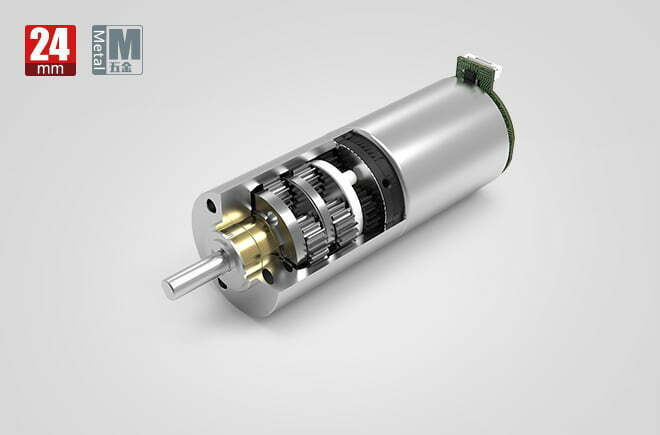# What is the meaning of planetary gearbox efficiency？

## What is the meaning of planetary gearbox efficiency?

The transmission efficiency of the planetary gearbox is determined by the gear stage output reduction ratio, the number of stages of the planetary gearbox by the sun wheel and its surrounding planetary wheel constitutes an independent reduction wheel system, such as the reducer only this one wheel system, we call “a section (level)” to get a greater reduction ratio, the need for multiple (level) transmission.

Since one set of planetary gears cannot meet the larger transmission ratio, sometimes two or three sets are needed to meet the user’s requirements for a larger transmission ratio. Since the number of planetary gears is increased, the length of two or three reduction stages will be increased and the efficiency will be reduced. The number of stages of planetary gearbox is different, the transmission efficiency is also different, usually not more than three, the smaller the number of stages the higher the transmission efficiency, and vice versa the smaller.One-stage (single-stage) planetary gearbox efficiency of 98%.
Second-stage planetary gearbox efficiency of 98% × 98%.
Three-stage planetary gearbox efficiency of 98% × 98% × 98%.
Full-load efficiency of planetary gear reducer: the transmission efficiency of planetary gear reducer under large load (fault stop output torque).

Planetary gear reducer efficiency calculation formula.
Planetary gearbox: the first stage: η = 0.90.9100% (normal ST28P\ST32P\ST36P\ST42P are calculated according to this)
56% (ST16P\ST22P low efficiency according to this calculation, the technical department needs to improve)
Multi-stage: η=η1*0.9 where n is the number of stages